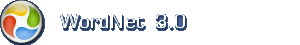# English dictionary

## English noun: calculation

1. calculation (act) the procedure of calculating; determining something by mathematical or logical methods

Synonymscomputation, computing

Narrower (hyponym)mathematical operation, mathematical process, number crunching, operation, recalculation, transposition

2. calculation (cognition) problem solving that involves numbers or quantities

Synonymscomputation, figuring, reckoning

Narrower (hyponym)approximation, conversion, derivative, derived function, differential, differential coefficient, estimate, estimation, extrapolation, first derivative, idea, integral, interpolation

3. calculation (cognition) planning something carefully and intentionally

SamplesIt was the deliberation of his act that was insulting.

Synonymsdeliberation